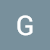# Introduction to Decision Tree Classifiers

Decision trees are a powerful tool capable of mapping non-linear relationships and complex relationships between many variables. They are also computationally inexpensive compared to other algorithms. There are many forms of decision trees, but I am going to just cover the basics of the algorithm in this post.

Decision trees…

# Checking The Assumptions For Linear Regression

In this post I will talk about the assumptions for linear regression. Some must be checked before modeling begins. Others need to be checked afterward using the residuals obtained from training. …

# Introduction To The Central Limit Theorem

When conducting statistical tests, many of them require that the values of the samples are normally distributed. Often times they are, unfortunately, not normally distributed, but there is a nifty trick to fix this problem. The solution involves what is called ‘The Central Limit Theorem’.

The central limit theorem states…

# Creating a Presentation for a Data Science Project

One of the most sought after skills in data analytics is to be able to tell a story with data. This is why you used the data visualizations, statistics, and machine learning, it was to influence business decisions. There should be plenty of domain knowledge put to use and you…

# Why Use Cross Validation?

Cross validation is an alternative to using a validation set for machine learning models. There are several ways using cross validation instead of a stand alone validation set can improve the performance of your models. …

# K-Neighbors From Scratch

Using just python and numpy

## Why use K-Neighbors?

K-Neighbors is a good at modeling complex non-linear relationships. It is a supervised form of machine learning and is different from linear and tree based algorithms because it is distance based.

## How Does K-Neighbors Work?

K-Neighbors estimates values by examining nearby data points. A K-Neighbors model works by first…

# An Introduction to the Pandas Library

By George Bennett

Pandas is a library in python that helps with organizing and exploring data. It is built on the numpy library. Pandas stores data in neat tables called “DataFrames” that can be easily manipulated. I will be explaining how to create dataframes, how to quickly get information from…

# The Differences Between Python Lists and Numpy Arrays

By George Bennett

If your learning python you will quickly become familiar with the list datatype. Lists are an ordered collection of data, whether it be numbers, strings, collections, or any other objects. Numpy arrays are similar to lists but when dealing with numerical information they simplify mathematical processes. …

# Scaling Data

By George Bennett

When using machine learning algorithms it is often a good idea to first scale the data. Scaling the data is putting all the features on a level playing field. Say you are using a distance based algorithm and you have one feature with a range in the…

# The Basics of Joining Tables

By George Bennett

Whether you are using tableau, SQL, or excel, or google sheets. It is a good Idea to know how to make joins.

Joins are used when you have two tables containing information that can be linked together. Many times in databases there will be identifier columns which…## Datascience George

Data scientist learning at Flat Iron School